#### The translational part

The state transition equation involves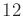scalar equations. Six of these are straightforward to obtain by characterizing the linear velocity. For this case, it can be imagined that the body does not rotate with respect to the inertial frame. The linear momentum is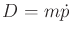, and Newton's second law implies that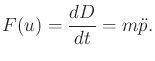(13.81)

This immediately yields half of the state transition equation by solving for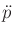. This yields a 3D version of the double integrator in Example 13.3, scaled by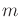. Let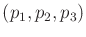denote the coordinates of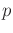. Let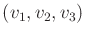denote the linear velocity the center of mass. Three scalar equations of the state transition equation are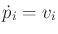for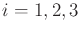. Three more are obtained as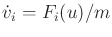for. If there are no moments and the body is not rotating with respect to the inertial frame, then these six equations are sufficient to describe its motion. This may occur for a spacecraft that is initially at rest, and its thrusters apply a total force only through the center of mass.

Steven M LaValle 2020-08-14